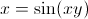## Test Prep Plan - Take a practice test

Take this practice test to check your existing knowledge of the course material. We'll review your answers and create a Test Prep Plan for you based on your results.
How Test Prep Plans work
1
2Based on your results, we'll create a customized Test Prep Plan just for you!
3Study smarter
Study more effectively: skip concepts you already know and focus on what you still need to learn.

# AP Calculus AB & BC: Tutoring Solution Final Exam

Free Practice Test Instructions:

Choose your answer to the question and click 'Continue' to see how you did. Then click 'Next Question' to answer the next question. When you have completed the free practice test, click 'View Results' to see your results. Good luck!

#### Question 2 2. Integrate the following.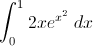#### Question 4 4. Using the graph, find the value of z, then add 1.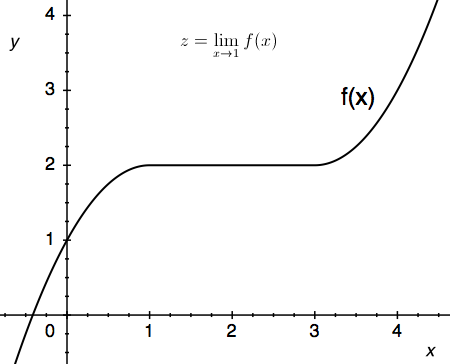#### Question 6 6. The lines a and b are parallel lines of length 5 and 2. The lines d and e are parallel to one another, and both are perpendicular to a and b. What is the length of c?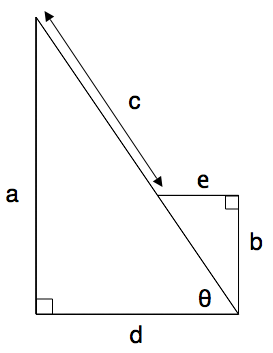#### Question 7 7. Which of the following graphs is the derivative of the graph below?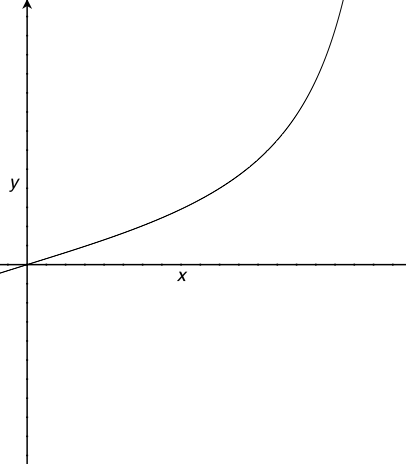#### Question 8 8. Given the graph of f '(x) below, what could the shape of look f(x) look like in the region between x = 0 and x = 4?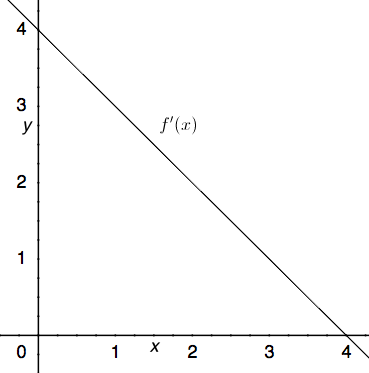#### Question 10 10. Which of the following is equivalent to the following expression?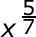#### Question 12 12. Consider the function below. According to the intermediate value theorem, is there a solution to f(x) = 0 for a value of x between -5 and 5?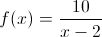#### Question 13 13. Which of the following BEST describes point B?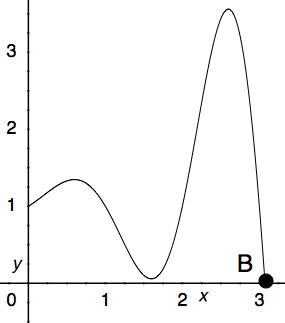#### Question 14 14. Which of the following radical expressions is equal to this exponential expression?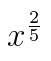#### Question 15 15. Find y'(x).